# Graph, bipartite

(diff) ← Older revision | Latest revision (diff) | Newer revision → (diff)

bichromatic graph

A graph whose setof vertices can be partitioned into two disjoint sets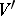and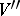(i.e.,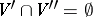) so that each edge connects some vertex ofwith some vertex of. A graph is bipartite if and only if all its simple cycles have even length. Another frequently used definition of a bipartite graph is a graph in which two subsetsandof vertices (parts) are given in advance. Bipartite graphs are convenient for the representation of binary relations between elements of two different types — e.g. the elements of a given set and a subset of it yield the relation of "membership of an element to a subset" , for executors and types of jobs one has the relation "a given executor can carry out a given job" , etc.

An important problem concerning bipartite graphs is the study of matchings, that is, families of pairwise non-adjacent edges. Such problems occur, for example, in the theory of scheduling (partitioning of the edges of a bipartite graph into a minimal number of disjoint matchings), in the problem of assignment (finding the maximum number of elements in a matching), etc. The cardinality of the maximum matching in a bipartite graph is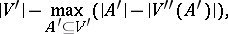where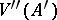is the number of vertices ofadjacent to at least one vertex of. A complete bipartite graph is a bipartite graph in which any two vertices belonging to different subsets are connected by an edge (e.g. the graph, see Graph, planar, Figure 1).

A generalization of the concept of a bipartite graph is the concept of a-partite graph, i.e. a graph in which the vertices are partitioned intosubsets so that each edge connects vertices belonging to different subsets.

How to Cite This Entry:
Graph, bipartite. Encyclopedia of Mathematics. URL: http://encyclopediaofmath.org/index.php?title=Graph,_bipartite&oldid=16372
This article was adapted from an original article by V.B. Alekseev (originator), which appeared in Encyclopedia of Mathematics - ISBN 1402006098. See original article